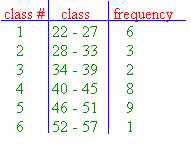### CFA Practice Question

There are 985 practice questions for this topic.

### CFA Practice Question

For the grouped frequency distribution shown below, the class width is ______.A. 18
B. 5
C. 6

The class width is the distance from the lower class limit to the lower class limit. Here, the class width is 28 - 22 = 6.

User Comment
chbourke Sorry. It is 5. Because is the clas width is the distance from the lower class limit to HIGH class limit. Here, the class width is 27 - 22 = 5 or 28 - 33 = 5
Rotigga No, 6 is correct. Just count: 22, 23, 24, 25, 26, 27-- that's six numbers.
gaur it is 6. Remember frequency should be non-exclusive and non-overlapping.
Sumit14 both the boundary values should be taken into account while counting
Ioannis The number of natural numbers between N and M where M>N is M-N+1 so 27-22+1=6
JKiro "interval" must be all-inclusive & non-overlapping
duongth307 Class width = (Highest - Lowest) / No. of class
= (57 - 22)/6 = 5.833 or 6 (as the nearest)
DutchNadja page 245 of the bible
ciji is this class war going on ?
Saxonomy **Throws pie in ciji's face**
Shaan23 Duongth....you're kind of correct....you have to use the Class Bounderies and NOT the class limits...you guys should see a question on class bounderies if you havent yet...

(57.5 - 21.5) / 6 = 6
Sm7272 Page 375 of the Bible :
Interval width = Range / no. Of intervals , rounded up.
(57-22)/6 = 35/6 which shall be rounded up.